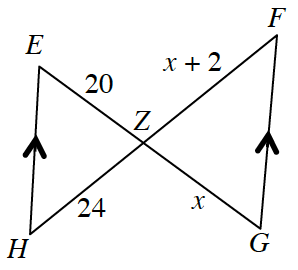### Home > INT2 > Chapter 4 > Lesson 4.1.2 > Problem4-22

4-22.

Examine the triangles in the diagram at right.

1. Are the triangles similar? If you decide that they are, then justify your conclusion using a flowchart.

What do the two arrows mean?
What do they tell you about angle relationships?

Yes, by $\text{AA~}$.
Draw a flowchart to display your conclusions.

2. Solve for $x$. Show all work.

Corresponding sides of similar triangles are proportional.

$\frac{20}{x}\ =\ \frac{_{\ \ \ \ \ \ \ \ \ \ \ }}{ }$